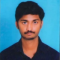## Factorize the following algebraic expressions:(i) \$y^2+5y-36\$(ii) \$(a^2-5a)^2-36\$(iii) \$(a+7)(a-10)+16\$Akhileshwar Nani

Updated on 11-Apr-2023 07:10:11

99 Views

Given:The given expressions are:(i) \$y^2+5y-36\$(ii) \$(a^2-5a)^2-36\$(iii) \$(a+7)(a-10)+16\$To do:We have to factorize the given algebraic expressions.Solution:Factorizing algebraic expressions:Factorizing an algebraic expression implies writing the expression as a product of two or more factors. Factorization is the reverse of distribution. An algebraic expression is factored completely when it is written as a product ... Read More

## Factorize the following algebraic expressions:(i) \$a^2+2a-3\$(ii) \$a^2+14a+48\$(iii) \$x^2-4x-21\$Akhileshwar Nani

Updated on 11-Apr-2023 07:08:46

58 Views

Given:The given expressions are:(i) \$a^2+2a-3\$(ii) \$a^2+14a+48\$(iii) \$x^2-4x-21\$To do:We have to factorize the given algebraic expressions.Solution:Factorizing algebraic expressions:Factorizing an algebraic expression implies writing the expression as a product of two or more factors. Factorization is the reverse of distribution. An algebraic expression is factored completely when it is written as a product ... Read More

## Factorize the following algebraic expressions:(i) \$x^2-22x+120\$(ii) \$x^2-11x-42\$Akhileshwar Nani

Updated on 11-Apr-2023 07:08:00

412 Views

Given:The given expressions are:(i) \$x^2-22x+120\$(ii) \$x^2-11x-42\$To do:We have to factorize the given algebraic expressions.Solution:Factorizing algebraic expressions:Factorizing an algebraic expression implies writing the expression as a product of two or more factors. Factorization is the reverse of distribution. An algebraic expression is factored completely when it is written as a product of ... Read More

## Factorize the following algebraic expressions:(i) \$a^2-8ab+16b^2-25c^2\$(ii) \$x^2-y^2+6y-9\$Akhileshwar Nani

Updated on 11-Apr-2023 07:07:02

62 Views

Given:The given expressions are:(i) \$a^2-8ab+16b^2-25c^2\$(ii) \$x^2-y^2+6y-9\$To do:We have to factorize the given algebraic expressions.Solution:Factorizing algebraic expressions:Factorizing an algebraic expression implies writing the expression as a product of two or more factors. Factorization is the reverse of distribution. An algebraic expression is factored completely when it is written as a product of prime ... Read More

## Factorize the following algebraic expressions:(i) \$25-p^2-q^2-2pq\$(ii) \$x^2+9y^2-6xy-25a^2\$(iii) \$49-a^2+8ab-16b^2\$Akhileshwar Nani

Updated on 11-Apr-2023 07:06:07

50 Views

Given:The given expressions are:(i) \$25-p^2-q^2-2pq\$(ii) \$x^2+9y^2-6xy-25a^2\$(iii) \$49-a^2+8ab-16b^2\$To do:We have to factorize the given algebraic expressions.Solution:Factorizing algebraic expressions:Factorizing an algebraic expression implies writing the expression as a product of two or more factors. Factorization is the reverse of distribution. An algebraic expression is factored completely when it is written as a product of prime ... Read More

## Factorize the following algebraic expressions:(i) \$4x^4+1\$(ii) \$4x^4+y^4\$Akhileshwar Nani

Updated on 11-Apr-2023 07:04:32

98 Views

Given:The given expressions are:(i) \$4x^4+1\$.(ii) \$4x^4+y^4\$To do:We have to factorize the given algebraic expressions.Solution:Factorizing algebraic expressions:Factorizing an algebraic expression implies writing the expression as a product of two or more factors. Factorization is the reverse of distribution. An algebraic expression is factored completely when it is written as a product of prime ... Read More

## Factorize the following algebraic expressions:(i) \$a^2+3a-88\$(ii) \$a^2-14a-51\$(iii) \$x^2+14x+45\$Akhileshwar Nani

Updated on 10-Apr-2023 22:44:03

108 Views

Given:The given expressions are:(i) \$a^2+3a-88\$(ii) \$a^2-14a-51\$(iii) \$x^2+14x+45\$To do:We have to factorize the given algebraic expressions.Solution:Factorizing algebraic expressions:Factorizing an algebraic expression implies writing the expression as a product of two or more factors. Factorization is the reverse of distribution. An algebraic expression is factored completely when it is written as a product ... Read More

## Factorize the following algebraic expressions:(i) \$x^2+12x-45\$(ii) \$40+3x-x^2\$Akhileshwar Nani

Updated on 10-Apr-2023 22:43:15

381 Views

Given:The given expressions are:(i) \$x^2+12x-45\$(ii) \$40+3x-x^2\$To do:We have to factorize the given algebraic expressions.Solution:Factorizing algebraic expressions:Factorizing an algebraic expression implies writing the expression as a product of two or more factors. Factorization is the reverse of distribution. An algebraic expression is factored completely when it is written as a product of ... Read More

## Factorize the following algebraic expressions:(i) \$49-x^2-y^2+2xy\$(ii) \$a^2+4b^2-4ab-4c^2\$(iii) \$x^2-y^2-4xz+4z^2\$Akhileshwar Nani

Updated on 10-Apr-2023 22:42:31

61 Views

Given:The given expressions are:(i) \$49-x^2-y^2+2xy\$(ii) \$a^2+4b^2-4ab-4c^2\$(iii) \$x^2-y^2-4xz+4z^2\$To do:We have to factorize the given algebraic expressions.Solution:Factorizing algebraic expressions:Factorizing an algebraic expression implies writing the expression as a product of two or more factors. Factorization is the reverse of distribution. An algebraic expression is factored completely when it is written as a product of prime ... Read More

## Factorize the following algebraic expressions:(i) \$25x^2-10x+1-36y^2\$(ii) \$a^2-b^2+2bc-c^2\$(iii) \$a^2+2ab+b^2-c^2\$Akhileshwar Nani

Updated on 10-Apr-2023 22:40:21

52 Views

Given:The given expressions are:(i) \$25x^2-10x+1-36y^2\$(ii) \$a^2-b^2+2bc-c^2\$(iii) \$a^2+2ab+b^2-c^2\$To do:We have to factorize the given algebraic expressions.Solution:Factorizing algebraic expressions:Factorizing an algebraic expression implies writing the expression as a product of two or more factors. Factorization is the reverse of distribution. An algebraic expression is factored completely when it is written as a product of prime ... Read More

Previous 1 ... 4 5 6 7 8 ... 21 Next